# Machine Vision Lenses Technical Terms

There are several types of imaging sensors for FA cameras, with different image sizes.The aspect ratio of FA camera is normally 4:3 (H:V).

Product symbol Image sensor Image size (mm)
Horizontal :H Vertical :V Diagonal :D
C 1” 12.8 9.6 16.0
H 2/3” 8.8 6.6 11.0
D 1/2” 6.4 4.8 8.0
35mm camera lens
(Reference)
35mm Film 36.0 24.0 43.3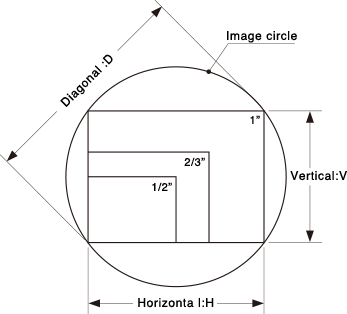The angle of view is the object size that can be captured at a specified image size,
which is represented by angular measure. Normally the angle of view is measured assuming a lens is focused at infinity. When using a lens of the same focal length with a different image size, the angle of view will differ.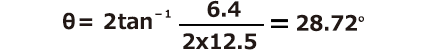Eg. The angle of view when the camera size is 1/2"
and the focal length is 12.5mm: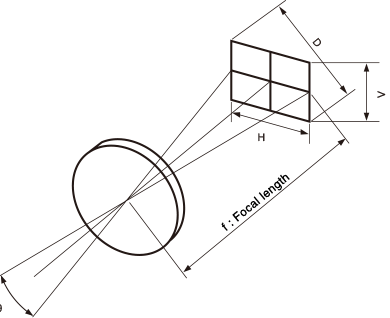When focusing on a certain area in front of and behind the deep object appears in focus. This area is called the depth of field. This is because the focus appears sharp if the focus misalignment is under a certain volume. This certain volume is called the permissible circle of confusion.

The depth of field has following properties.

• 1)The larger the F No. is, the wider the depth of field becomes.

• 2)The shorter the focal length is, the wider the depth of field becomes.

• 3)The longer the distance to the object is, the wider depth of field becomes.

• 4)The backward depth of field is wider than the forward depth of field.
Image sensor Permissible circle of confusion
1” 0.03 mm
2/3” 0.021 mm
1/2” 0.015 mm
1/3” 0.011 mm
1/4” 0.008 mm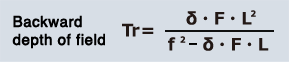The depth of field can be calculated by the following formula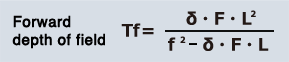Depth of field ＝
Tr＋Tf
Focal depth ＝
2δ・F

f ： Focal distance
F ： F No.
δ ： Permissible circle diameter of confusion
L ： Object distance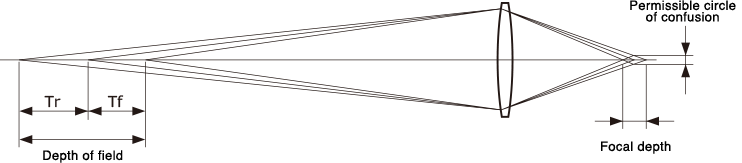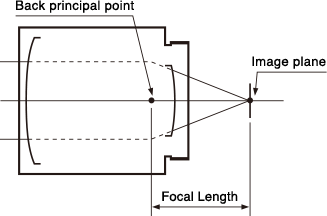The focal length will be the distance from the back principal point to the image plane.
Lower the focal length wider the imageThe F No. is an indication of the brightness of lens. The smaller the value, the brighter the image produced by the lens. The F No. is inversely proportional to the effective diameter of the lens and directly proportional to the focal length.
The F No. is a value determined on the assumption that the transmittance of the lens is 100%.
Virtually all lenses however, have different spectral transmittance, and thus, the same F No. can have different levels of brightness.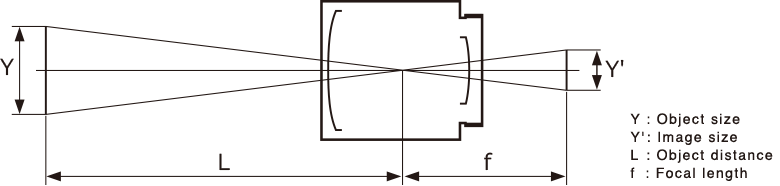(1) How to calculate the field of view
If the distance to the object is finite, you can use the following formula to calculate the field of view.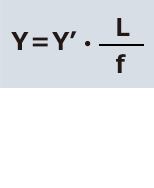Eg. 1/3" CCD camera with an 8mm lens is used, and the distance to the object is 3m. The maximum horizontal width as viewed on the monitor can be calculated as follows.

• Y':　4.8
• L:　3000
• f:　8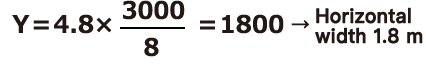(2) How to calculate focal length
If the distance to the object is finite, you can use the following formula to calculate the focal length.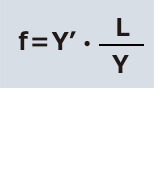Eg.1/3" CCD camera with an 8mm lens is used, and the distance to the object is 3m. The maximum horizontal width as viewed on the monitor can be calculated as follows.

• Y':　4.8
• L:　3000
• f:　8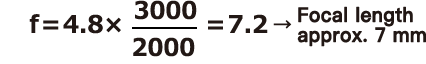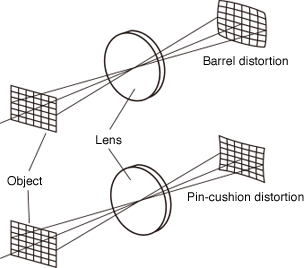Distortion is an aberration where the geometric figure of the object is not reproduced faithfully at the image plane. It is normally represented by the level shift of an image point from its ideal position by a percentage of image height or width.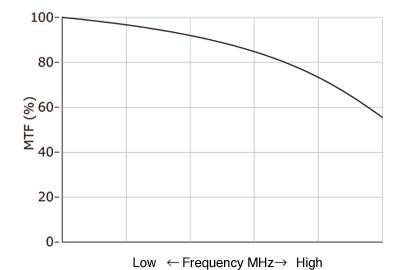MTF (Modulation Transfer Function) represents the declining contrast rate when shooting a chart consisted of black and white lines.